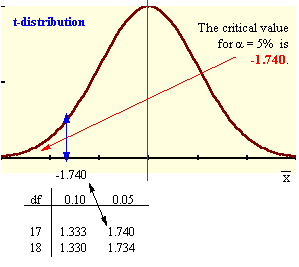### CFA Practice Question

There are 985 practice questions for this topic.

### CFA Practice Question

A hypothesis test is constructed at the 5% level of significance where the population standard deviation is unknown. A random sample of size n = 18 yields a sample mean of 67 and a sample standard deviation of 6. The critical value for H0: μ > 70 is ______.A. -1.740
B. -1.645
C. 1.645

Because the population standard deviation, σ, is unknown, we use a t-distribution: t(0.05, 18 - 1). Looking in the t-table under df = 17 and under column 0.05, we get 1.740. The rejection region is to the bottom 5%, so the critical value is -1.740.